Courses
Courses for Kids
Free study material
Free LIVE classes
More

# 1 Digit Multiplication## Introduction to 1 Digit by 1 Digit Multiplication Worksheets PDF

The repeated addition of one number in relation to another is represented by the multiplication of two numbers. In mathematics, multiplying is equivalent to adding equal groups. As we multiply, the number of things in the group increases. The product, the two factors, and the product are all components of a multiplication problem.

## What are 1 Digit Numbers?

We are aware that the digits 1, 2, 3, 4, 5, 6, 7, 8, 9 and 0 are used to create all numbers. One digit, two digits, and even several digits can be used to construct various numerals. There are 9 single-digit numbers. 1 (one) is the smallest and 9 (nine) is the largest one-digit number. When used as a number, all the digits are converted to numbers.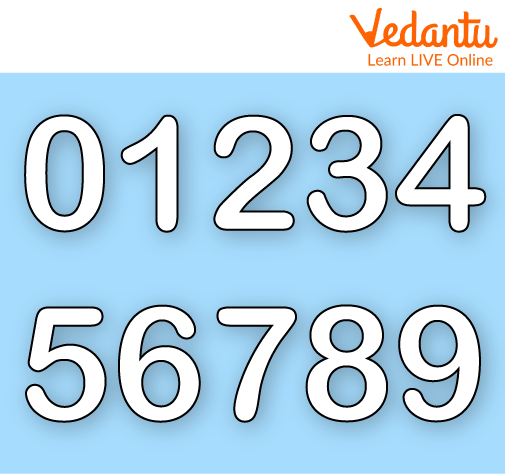Numbers

## What is Multiplication?

Multiplication in mathematics is the same as adding equal groups. The number of things in the group grows as we multiply. A multiplication problem has three parts: the product, the two factors, and the product. These figures can be fractions, integers, whole numbers, natural numbers, etc. When a is multiplied by b, either a is added to itself 'b' times or the other way around. In mathematics, besides addition, subtraction, and division, multiplication is a mathematical operation symbolised by the symbol "✖ ".

## Important Terminologies Used in Multiplication:

The "factors" are often the numbers that need to be multiplied. Example 2 is a factor of 4. The "multiplicand" is the number that has to be multiplied, and the "multiplier" is the number by which it is multiplied. Normally, the multiplier comes first and the multiplicand comes second; however, sometimes the multiplicand comes first and the multiplier comes second.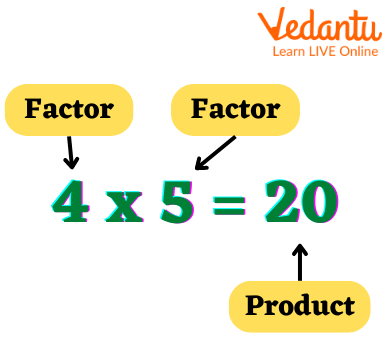Different Terms of Multiplication

## Properties of Multiplication :

The properties of multiplication are certain rules that are used while multiplying numbers.

• Commutative property of multiplication: The product is unaffected by the order of the multiplier and multiplicand.

For instance, $3 \times 4 = 12$and $4 \times 3 = 12$Thus, the result remains unaffected.

• Associative property of multiplication: It states that altering the way the elements are grouped has no effect on the result.

For instance, $[3 \times 9] \times 2 = 3 \times [9 \times 2]$

• Identity property of multiplication: It states that whenever we multiply any number with 1 we will get the number itself.

For instance, $8 \times 1 = 8$

## Multiplication Steps:

From right to left, multiply the multiplicand by each of the multiplier's digits. Below the line, the product's units are written.

Each product's tenth position is added to the product after it.

For Instance: , if we take $561 \times 3$ :

The first calculation is $3 \times 1 = 3$. The 3 is entered beneath the line.

Now, multiply 3 by 6 to get 18. The 1 from the tens place is saved and added to the subsequent multiplication once the eight is written below the line.

To finish, multiply $3 \times 5$to get 15. The 1 we saved from the previous product must be added on, making the total $15 + 1 = 16$. Nothing else needs to be done.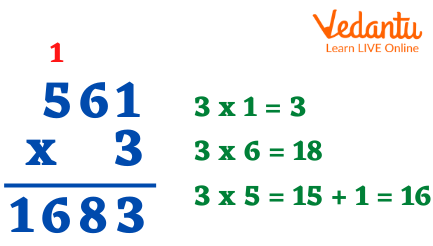Multiplication

## One Digit Multiplication:

We are familiar with how to multiply one 1-digit number by one more 1-digit number. The multiplication table for the relevant integer is used when multiplying one-digit numbers.

Let's say we need to multiply 6 by 4 and we arrange the numbers in a column.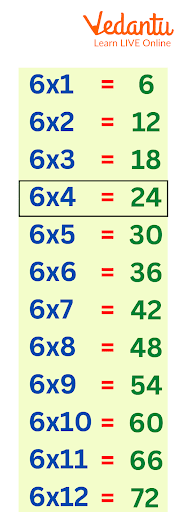Multiplication

To determine that 6 times 4 are 24, we must mentally read the multiplication tables of 6.

24 is listed as a product. We state that 6 times 4 equals 24.

In this case, the multiplicand is 6, the multiplier is 4, and the multiple fact of the product is 24.

The numbers 6 and 4 are frequently referred to as the 24's component.

## Multiplication with Zero:

Zero has an additive identity because it can be added to any number without changing its identity. The zero property of multiplication states that the product of multiplying a number by zero is always zero. Because the zero can come before or after the number, the position of the zero has no effect on the property.

So, $5 \times 0 = 0$. The zero property of multiplication applies to all numbers, including integers, fractions, decimals, and algebraic terms.

As a result, zero times any numerical digit equals zero.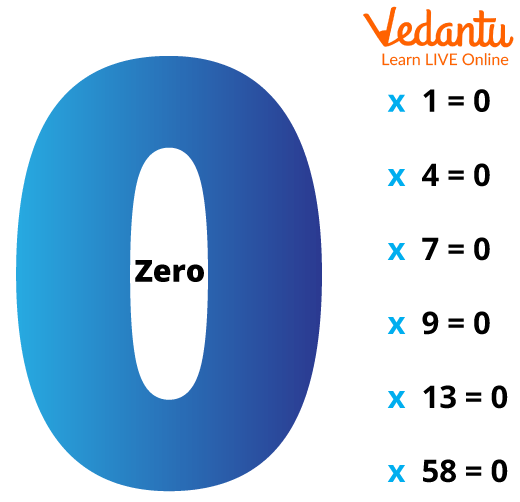Multiplication with Zero

## Solved Examples(One Digit Multiplication Worksheets) :

1: What will 9 times 7 equals ?

Ans: 9 times 7 can be written as :

$9 \times 7=63$

9 times 7 equals 63.

2: Find the value for 0 * 0.

Ans: The value for 0 * 0 will be 0.

As we know that if we multiply any number (here it is 0) with 0 we will get 0.

3: If a child gets 8 chocolates every day, then how many chocolates will he have in a week?

Ans: We know that 1 week $= 7$ days

And child gets 8 chocolates every day

Means after 1 week he will have $8 \times 7$ chocolates .

$8 \times 7=56$

After 1 week he will have 56 chocolates with him.

## Conclusion :

Mathematical multiplication is equivalent to adding equal groups. As we multiply, the number of things in the group increases. The product, the two factors, and the product are the three components of a multiplication problem. Multiplication is represented by the symbol "✖".

Last updated date: 24th Sep 2023
Total views: 80.4k
Views today: 0.80k

## FAQs on 1 Digit Multiplication

1. Who brought the concept of multiplication?

The concept of multiplication was brought up by the famous mathematician, The Babylonians. Nearly, four thousand years ago, the Babylonians gave the concept of multiplication.

2. What are the other symbols for multiplication?

The other symbols are: (⋅),  (∗) between two mathematical expressions, denoting multiplication of the second expression by the first.

3. Is there any sequence to learn multiplication tables?

Yes, each multiplication table has a different sequence of numbers. Like in the multiplication table of 2, there is a skip counting of 2 digits.